# plot¶

Plot lines, polygons, and symbols in 2-D

## Synopsis¶

gmt plot [ table ] -Jparameters -Rwest/east/south/north[/zmin/zmax][+r][+uunit] [ -A[m|p|x|y|r|t] ] [ -B[p|s]parameters ] [ -Ccpt ] [ -Ddx/dy ] [ -E[x|y|X|Y][+a|A][+cl|f][+n][+wcap][+ppen] ] [ -F[c|n|r][a|f|s|r|refpoint] ] [ -Gfill|+z ] [ -H[scale] ] [ -I[intens] ] [ -L[+b|d|D][+xl|r|x0][+yl|r|y0][+ppen] ] [ -N[c|r] ] [ -S[symbol][size] ] [ -T ] [ -U[stamp] ] [ -V[level] ] [ -W[pen][attr] ] [ -X[a|c|f|r][xshift] ] [ -Y[a|c|f|r][yshift] ] [ -Zvalue|file] [ -aflags ] [ -bibinary ] [ -di[+ccol]nodata ] [ -eregexp ] [ -fflags ] [ -ggaps ] [ -hheaders ] [ -iflags ] [ -lflags ] [ -pflags ] [ -qiflags ] [ -ttransp ] [ -wflags ] [ -:[i|o] ] [ --PAR=value ]

## Description¶

Reads (x,y) pairs from files [or standard input] and will plot lines, polygons, or symbols at those locations on a map. If a symbol is selected and no symbol size given, then it will interpret the third column of the input data as symbol size. Symbols whose size is <= 0 are skipped. If no symbols are specified then the symbol code (see -S below) must be present as last column in the input. If -S is not used, a line connecting the data points will be drawn instead. To explicitly close polygons, use -L. Select a fill with -G. If -G is set, -W will control whether the polygon outline is drawn or not. If a symbol is selected, -G and -W determines the fill and outline/no outline, respectively.

## Required Arguments¶

table

One or more ASCII (or binary, see -bi[ncols][type]) data table file(s) holding a number of data columns. If no tables are given then we read from standard input.

-Jparameters

Specify the projection. (See full description) (See cookbook summary) (See projections table).

-Rxmin/xmax/ymin/ymax[+r][+uunit]

Specify the region of interest. Note: If using modern mode and -R is not provided, the region will be set based on previous plotting commands. If this is the first plotting command in the modern mode levels and -R is not provided, the region will be automatically determined based on the data in table (equivalent to using -Ra). (See full description) (See cookbook information).

For perspective view -p, optionally append /zmin/zmax. (more …)

## Optional Arguments¶

-A[m|p|x|y|r|t]

By default, geographic line segments are drawn as great circle arcs by resampling coarse input data along such arcs. To disable this sampling and draw them as straight lines, use the -A flag. Alternatively, add m to draw the line by first following a meridian, then a parallel. Or append p to start following a parallel, then a meridian. (This can be practical to draw a line along parallels, for example). For Cartesian data, points are simply connected, unless you append x or y to draw stair-case curves that whose first move is along x or y, respectively. For polar projection, append r or t to draw stair-case curves that whose first move is along r or theta, respectively.

-B[p|s]parameters

Set map boundary frame and axes attributes. (See full description) (See cookbook information).

-Ccpt

Give a CPT or specify -Ccolor1,color2[,color3,…] to build a linear continuous CPT from those colors automatically, where z starts at 0 and is incremented by one for each color. In this case colorn can be a r/g/b triplet, a color name, or an HTML hexadecimal color (e.g. #aabbcc ). If -S is set, let symbol fill color be determined by the z-value in the third column. Additional fields are shifted over by one column (optional size would be 4th rather than 3rd field, etc.). If -S is not set, then it expects the user to supply a multisegment file where each segment header contains a -Zval string. The val will control the color of the line or polygon (if -L is set) via the CPT. If modern mode and no argument is given then we select the current CPT.

-Ddx/dy

Offset the plot symbol or line locations by the given amounts dx/dy [Default is no offset]. If dy is not given it is set equal to dx. You may append dimensional units from c|i|p to each value.

-E[x|y|X|Y][+a|A][+cl|f][+n][+wcap][+ppen]

Draw symmetrical error bars. Append x and/or y to indicate which bars you want to draw (Default is both x and y). The x and/or y errors must be stored in the columns after the (x,y) pair [or (x,y,z) triplet]. If +a is appended then we will draw asymmetrical error bars; these requires two rather than one extra data column, with the two signed deviations. Use +A to read the low and high bounds rather than signed deviations. If upper case X and/or Y are used we will instead draw “box-and-whisker” (or “stem-and-leaf”) symbols. The x (or y) coordinate is then taken as the median value, and four more columns are expected to contain the minimum (0% quantile), the 25% quantile, the 75% quantile, and the maximum (100% quantile) values. The 25-75% box may be filled by using -G. If +n is appended the we draw a notched “box-and-whisker” symbol where the notch width reflects the uncertainty in the median. This symbol requires a 5th extra data column to contain the number of points in the distribution. The +w modifier sets the cap width that indicates the length of the end-cap on the error bars [7p]. Pen attributes for error bars may also be set via +ppen. [Defaults: width = default, color = black, style = solid]. When -C is used we can control how the look-up color is applied to our symbol. Append +cf to use it to fill the symbol, while +cl will just set the error pen color and turn off symbol fill. Giving +c will set both color items. Note: The error bars are placed behind symbols except for the large vertical and horizontal bar symbols (-Sb|B) where they are plotted on top to avoid the lower bounds being obscured.

-F[c|n|p][a|f|s|r|refpoint]

Alter the way points are connected (by specifying a scheme) and data are grouped (by specifying a method). Append one of three line connection schemes: c: Draw continuous line segments for each group [Default]. p: Draw line segments from a reference point reset for each group. n: Draw networks of line segments between all points in each group. Optionally, append the one of four segmentation methods to define the group: a: Ignore all segment headers, i.e., let all points belong to a single group, and set group reference point to the very first point of the first file. f: Consider all data in each file to be a single separate group and reset the group reference point to the first point of each group. s: Segment headers are honored so each segment is a group; the group reference point is reset to the first point of each incoming segment [Default]. r: Same as s, but the group reference point is reset after each record to the previous point (this method is only available with the -Fp scheme). Instead of the codes a|f|s|r you may append the coordinates of a refpoint which will serve as a fixed external reference point for all groups.

-Gfill|+z (more …)

Select color or pattern for filling of symbols or polygons [Default is no fill]. Note that this module will search for -G and -W strings in all the segment headers and let any values thus found over-ride the command line settings. If -Z is set, use -G+z to assign fill color via -Ccpt and the z-values obtained. Finally, if fill = auto[-segment] or auto-table then we will cycle through the fill colors implied by COLOR_SET and change on a per-segment or per-table basis. Any transparency setting is unchanged.

-H[scale]

Scale symbol sizes and pen widths on a per-record basis using the scale read from the data set, given as the first column after the (optional) z and size columns [no scaling]. The symbol size is either provided by -S or via the input size column. Alternatively, append a constant scale that should be used instead of reading a scale column.

-Iintens

Use the supplied intens value (nominally in the -1 to +1 range) to modulate the fill color by simulating illumination [none]. If no intensity is provided we will instead read intens from the first data column after the symbol parameters (if given).

-L[+b|d|D][+xl|r|x0][+yl|r|y0][+ppen] Example
X

## -L example

cat << EOF > t.txt
1 1
2 3
3 2
4 4
EOF
gmt begin filler pdf
gmt plot -R0/5/0/5 -JX3i -B t.txt -Gred -W2p -L+yb
gmt plot -B t.txt -Gred -W2p -L+yt -X3.25i
gmt plot -B t.txt -Gred -W2p -L+xl -X-3.25i -Y3.25i
gmt plot -B t.txt -Gred -W2p -L+xr -X3.25i
gmt plot -B t.txt -Gred -W2p -L+y4 -X-3.25i -Y3.25i
gmt plot -B t.txt -Gred -W2p -L+x4.5 -X3.25i
gmt end show

Force closed polygons. Alternatively, append modifiers to build a polygon from a line segment. Append +d to build symmetrical envelope around y(x) using deviations dy(x) given in extra column 3. Append +D to build asymmetrical envelope around y(x) using deviations dy1(x) and dy2(x) from extra columns 3-4. Append +b to build asymmetrical envelope around y(x) using bounds yl(x) and yh(x) from extra columns 3-4. Append +xl|r|x0 to connect first and last point to anchor points at either xmin, xmax, or x0, or append +yb|t|y0 to connect first and last point to anchor points at either ymin, ymax, or y0. Polygon may be painted (-G) and optionally outlined by adding +ppen [no outline]. Note: When options like -G and -Z are passed via segment headers you will need -L to ensure your segments are interpreted as polygons.

-N[c|r]

Do NOT clip symbols that fall outside map border [Default plots points whose coordinates are strictly inside the map border only]. The option does not apply to lines and polygons which are always clipped to the map region. For periodic (360-longitude) maps we must plot all symbols twice in case they are clipped by the repeating boundary. The -N will turn off clipping and not plot repeating symbols. Use -Nr to turn off clipping but retain the plotting of such repeating symbols, or use -Nc to retain clipping but turn off plotting of repeating symbols.

-S[symbol][size]

Plot symbols (including vectors, wedges, fronts, decorated or quoted lines). If present, size is symbol size in the default unit set by PROJ_LENGTH_UNIT (unless c, i, or p is appended). If the symbol code (see below) is not given it will be read from the last column in the input data; this scheme cannot be used in conjunction with binary input. Optionally, append c, i, or p to indicate that the size information in the input data is in units of cm, inch, or point, respectively [Default is PROJ_LENGTH_UNIT]. Note: If you provide both size and symbol via the input file you must use PROJ_LENGTH_UNIT to indicate the unit used for the symbol size or append the units to the sizes listed in the file. If symbol sizes are expected via one or more data columns then you may convert those values to suitable symbol sizes via the -i mechanism. The general input expectations are:

coordinates [ value ] [ parameters ] [ symbol ]

where coordinates is two or three columns specifying the location of a point, the optional value is required when -C is used to control color, the optional parameters is required when no symbol size is specified, and the trailing text with leading symbol code is required when the symbol code is not specified on the command line. Note: parameters may represent more than one size column as some symbols require several parameters to be defined (e.g., a circle just needs one column, a rectangle needs two dimensions, while an ellipse needs an orientation and two dimensions, and so on); see specifics below. When there is only a single parameter we will refer to it as size.

You can also change symbols by adding the required -S option to any of your multi-segment headers.

We will first outline the 14 basic geometric symbols that only require a single parameter: size.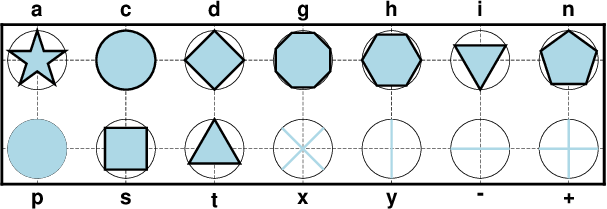The 14 basic geometric symbols available, shown with their symbol codes. Four symbols (-|+|x|y) are line-symbols only (-W), one (the point p only takes a color via -G) while the rest may have outline (-W) and fill (-G) specified. The thin circles represent the circumscribing circle of the same size.¶
-S-size

x-dash (-). size is the length of a short horizontal (x-dir) line segment.

-S+size

Plus (+). size is diameter of circumscribing circle.

-Sasize

Star. size is diameter of circumscribing circle.

-Scsize

circle. size is diameter of circle.

-Sdsize

diamond. size is diameter of circumscribing circle.

-Sgsize

Octagon. size is diameter of circumscribing circle.

-Shsize

hexagon. size is diameter of circumscribing circle.

-Sisize

inverted triangle. size is diameter of circumscribing circle.

-Snsize

Pentagon. size is diameter of circumscribing circle.

-Spsize

point. No size needs to be specified (1 pixel is used).

-Sssize

square. size is diameter of circumscribing circle.

-Stsize

triangle. size is diameter of circumscribing circle.

-Sxsize

Cross (x). size is diameter of circumscribing circle.

-Sysize

y-dash (|). size is the length of a short vertical (y-dir) line segment.

Note: The uppercase symbols A, C, D, G, H, I, N, S, T are normalized to have the same area as a circle with diameter size, while the size of the corresponding lowercase symbols refers to the diameter of a circumscribed circle.

The next collection shows five symbols that require two or more parameters, and some have optional modifiers to enhance the symbol appearance.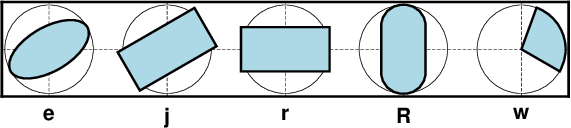Five basic geometric multi-parameter symbols, shown with their symbol codes. All take two or three parameters to define the symbol; see below for details. Upper-case versions E, J, and W are similar to e, j and w but expect geographic azimuths and distances.¶
-Sedirection/major_axis/minor_axis

ellipse. If not given, then direction (in degrees counter-clockwise from horizontal), major_axis, and minor_axis must be found after the coordinates [and value] columns. This option yields a Cartesian ellipse whose shape is unaffected by the map projection. If only a single size is given then we plot a degenerate ellipse (circle) with given diameter.

-SEazimuth/major_axis/minor_axis

Same as -Se, except azimuth (in degrees east of north) should be given instead of direction. The azimuth will be mapped into an angle based on the chosen map projection (-Se leaves the directions unchanged.) Furthermore, major_axis and minor_axis must be given in geographical instead of plot-distance units. For degenerate ellipses (i.e., circles) with just a diameter given via the input data, use -SE-. For a linear projection we assume the dimensions are given in the same units as -R. For allowable geographical units, see Units and append desired unit to the dimension(s) [Default is k for km]. The shape of the ellipse will be affected by the properties of the map projection.

-Sjdirection/width/height

Rotated rectangle. If not given, then direction (in degrees counter-clockwise from horizontal), width, and height must be found after the location [and value] columns. If only a single size is given then we plot a degenerate rectangle (square) with given size.

-SJazimuth/width/height

Same as -Sj, except azimuth (in degrees east of north) should be given instead of direction. The azimuth will be mapped into an angle based on the chosen map projection (-Sj leaves the directions unchanged.) Furthermore, the two dimensions must be given in geographical instead of plot-distance units. For a degenerate rectangle (i.e., square) with one dimension expected to be given via the input data, use -SJ-. For a linear projection we assume the dimensions are given in the same units as -R. For allowable geographical units, see Units and append desired unit to the dimension(s) [Default is k for km]. The shape of the rectangle will be affected by the properties of the map projection.

-Srwidth/height

rectangle. If width/height are not given, then these dimensions must be found after the location [and value] columns. Alternatively, append +s and then the diagonal corner coordinates are expected after the location [and value] columns instead.

Rounded rectangle. If width/height/radius are not given, then the two dimensions and corner radius must be found after the location [and value] columns.

-Sw[outer[/startdir/stopdir]][+i[inner]]

Pie wedge. Give the outer diameter outer, startdir and stopdir. These are directions (in degrees counter-clockwise from horizontal) for wedge. Parameters not appended are read from file after the location [and value] columns. Append +i and append a nonzero inner diameter inner or it will be read last . Append +a[dr] to draw the arc line (at inner and outer diameter); if dr is appended then we draw all arc lines separated radially by dr. Append +r[da] to draw radial lines (at start and stop directions) if da is appended then we draw all radial lines separated angularly by da. These spider-web lines are drawn using the current pen unless +ppen is added.

-SW[outer[/startaz/stopaz]][+i[inner]]

Same as -Sw, except azimuths (in degrees east of north) should be given instead of the two directions. The azimuths will be mapped into angles based on the chosen map projection (-Sw leaves the directions unchanged). The two diameters are expected in geographic units. However, if specified on the command line we also accept plot dimension units. Append +i and append a nonzero inner diameter inner or it will be read last . Append +a[dr] to draw the arc line (at inner and outer diameter); if dr is appended then we draw all arc lines separated radially by dr. Append +r[da] to draw radial lines (at start and stop directions) if da is appended then we draw all radial lines separated angularly by da. These spider-web lines are drawn using the current pen unless +ppen is added. For allowable geographical units, see Units [Default is k for km].

Text is normally placed with text but there are times we wish to treat a character of even a string as a symbol to be plotted: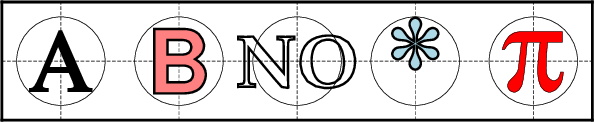A text symbol can be any letter or string (up to 256 characters) and you may specify specific fonts (size and type) and control outline and the fill properties. Note there is no mechanism to perfectly center the string; see -D to make a simple global adjustment.¶
-Slsize+tstring[+ffont][+jjustify]

letter or text string (less than 256 characters). Give size, and append +tstring after the size. Note: The size is only approximate; no individual scaling is done for different characters. Remember to escape special characters like *. Optionally, you may append +ffont to select a particular font [Default is FONT_ANNOT_PRIMARY] and +jjustify to change justification [CM].

The next type of symbol is the horizontal or vertical bar: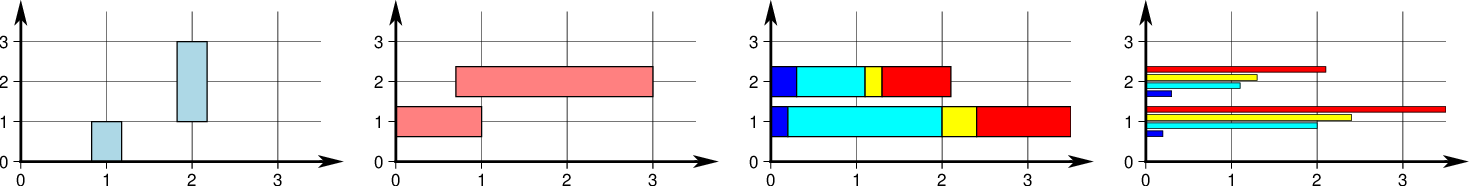We may place vertical (b) or horizontal (B) bars, and they may extend from the base of your choosing. The thickness of a bar can be a given dimension or can be specified in the units of that axis so its width scales with the projection and region. Using modifiers +v or +i you can also plot multi-band bars with colors set via -C, and +s can represent those as groups of individual bars instead.¶
-Sb[size[c|i|p|u]][+b|B[base]][+v|iny][+s[gap]]

Vertical bar extending from base to y. The size is bar width. Append u if size is in x-units [Default is plot-distance units]. By default, base = 0. Append +b[base] to change this value. If base is not appended then we read it from the last input data column. Use +B[base] if the bar height is measured relative to base [Relative to origin]. Normally, the bar requires a single input y-value. To plot multi-band bars, please append +v|iny, where ny indicates the total number of bands in the bar. Here, +i means we must accumulate the bar values from the increments dy, while +v means we get the complete values relative to base. Normally, the bands are plotted as sections of a final single bar. Use +s to instead split the bar into ny side-by-side, individual and thinner bars. The optional gap is a percent (of fraction) of size for adding gaps between the bars [none], where size is the combined width of all the individual, thinner bars plus the gaps. Multiband bars requires -C with one color per band (CPT z-values must be 0, 1, …, ny - 1). Thus, input records are either (x y1 y2 … yn) or (x dy1 dy2 … dyn).

-SB[size[c|i|p|u]][+b|B[base]][+v|inx][+s[gap]]

Horizontal bar extending from base to x. The size is bar width. Append u if size is in y-units [Default is plot-distance units]. By default, base = 0. Append +b[base] to change this value. If base is not appended then we read it from the last input data column. Use +B[base] if the bar length is measured relative to base [Relative to origin]. Normally, the bar requires a single input x-value. To plot multi-band bars, please append +v|inx, where nx indicates the total number of bands in the bar. Here, +i means we must accumulate the bar value from the increments dx, while +v means we get the complete values relative to base. Normally, the bands are plotted as sections of a final single bar. Use +s to instead split the bar into nx side-by-side, individual and thinner bars. The optional gap is a percent (of fraction) of size for adding gaps between the bars [none], where size is the combined width of all the individual, thinner bars plus the gaps. Multiband bars requires -C with one color per band (CPT z-values must be 0, 1, …, nx - 1). Thus, input records are either (x1 y x2 … xn) or (dx1 y dx2 … dxn).

The next family of symbols are all different types of vectors. Apart from requiring parameters such as length and direction (or optionally the coordinates of the end point), we also offer a rich set of modifiers to customize the vector head(s).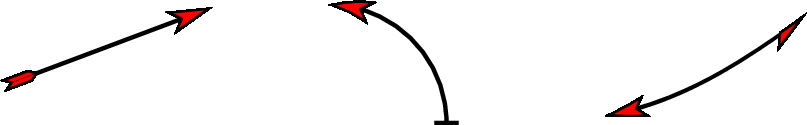There are three classes of vectors: Cartesian (left), circular (center) and geographic (right). While their use is slightly different, they all share common modifiers that affect how they are displayed.¶
-Smsize[+vecmodifiers]

math angle arc, optionally with one or two arrow heads [Default is no arrow heads]. The size is the length of the vector head. Arc width is set by -W, with vector head outlines defaulting to half of arc width. The radius of the arc and its start and stop directions (in degrees counter-clockwise from horizontal) must be given after the location [and value] columns. See Vector Attributes for specifying other attributes.

-SMsize[+vecmodifiers]

Same as -Sm but switches to straight angle symbol if start and stop angles subtend 90 degrees exactly.

-Svsize[+vecmodifiers]

vector. The direction (in degrees counter-clockwise from horizontal) and length must be found after the location [and value] columns, and size, if not specified on the command-line, should be present as well, pushing the other items to later columns. The size is the length of the vector head. Vector stem width is set by -W, with head outline pen width defaulting to half of stem pen width. See Vector Attributes for specifying this and other attributes.

-SVsize[+vecmodifiers]

Same as -Sv, except azimuth (in degrees east of north) should be given instead of direction. The azimuth will be mapped into an angle based on the chosen map projection (-Sv leaves the directions unchanged.) If your length is not in plot units but in arbitrary user units (e.g., a rate in mm/yr) then you can use the -i option to scale the corresponding column via the +sscale modifier. See Vector Attributes for specifying symbol attributes.

-S=size[+vecmodifiers]

Geographic vector. Here, azimuth (in degrees east from north) and geographical length must be found after the location [and value] columns. The size is the length of the vector head. Vector width is set by -W. See Vector Attributes for specifying attributes. Note: Geovector stems are drawn as thin filled polygons and hence pen attributes like dashed and dotted are not available. For allowable geographical units, see Units.

The next symbol is the programmable custom symbol: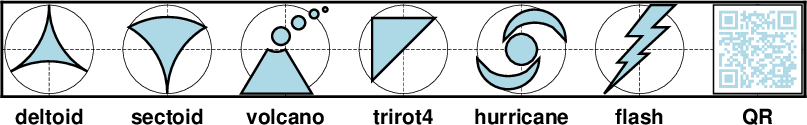Custom symbols are designed using the Custom Symbol Macro Language. We supply about 40 custom symbols, and users have contributed discipline-specific symbols for structural geology and marine biology that you can explore from the RESOURCES page (see sidebar), so you have lots of examples to use as a template. The language allows you to design symbols that takes many parameters and can make decisions based on these parameters.¶
-Skname/size

## GMT4 Vector¶

Note: The old-style, single-polygon vector available in GMT4 and earlier versions has been added to GMT5 for backwards compatibility with old scripts. For reference, the old vector syntax is listed here: The size, if present, will be interpreted as arrowwidth/headlength/headwidth. By default, arrow attributes remain invariant to the length of the arrow. To have the size of the vector scale down with decreasing length, append nnorm, so that vectors shorter than norm will have their dimensions scaled by the vector length divided by norm. To center the vector on the balance (mid) point, use -Svb; to align point with the vector head, use -Svh; to align point with the vector tail, use -Svt [Default]. If the input has the head point’s coordinates instead of direction and length, use -Svs. Upper case B, H, T, or S will draw a double-headed vector [Default is single head].

## Polar Caps¶

We will automatically determine if a closed polygon is containing a geographic pole, i.e., being a polar cap. Such polygons requires special treatment under the hood to ensure proper filling. Many tools such as GIS packages are unable to handle polygons covering a pole and some cannot handle polygons crossing the Dateline. They work around this problem by splitting polygons into a west and east polygon or inserting artificial helper lines that makes a cut into the pole and back. Such doctored polygons may be misrepresented in GMT.

## Bezier spline¶

The +s modifier to pen settings (-W) is limited to plotting lines and polygons. Lines with embellishments (fronts, decorated, or quoted lines) are excluded.

## Auto-Legend¶

The -l option for symbols expects the symbol color, size, and type to be given on the command line. If you have variable symbol sizes then you must append +ssize to set a suitable size for the legend entry. For other symbol cases the -l option will be ignored. Legend entries also work for lines and polygons, but not more complicated features such as decorated and quoted lines, fronts, etc.

## Data Column Order¶

The -S option determines how many size columns are required to generate the selected symbol, but if size is not given then we expect to read size from file. In addition, your use of options -H, -I and -t will require extra columns. The order of the data record is fixed regardless of option order, even if not all items may be activated. We expect data columns to come in the following order:

x y [z] [size-columns] [scale] [intens] [transp [transp2]] [trailing-text]


where items given in brackets are optional and under the control of the stated options: -C selects the optional z column for color-lookup, -S without a size selects the optional size-columns (may be more than one depending on the selected symbol), -H selects the optional scale column, -I selects the optional intens column, and -t selects the optional transp column(s). Trailing text is always optional. Notes: (1) If symbol code is not given by -S then it is expected to start the trailing text. (2) You can use -i to rearrange your data record to match the expected format.

## Auto-legend entries¶

This module allows you to use the -l option to specify an automatic legend entry. This option is available for lines or symbols only. If there is no size specified (which is always true for lines) or if the symbol size is computed from other information (which may be true for some symbols deriving size from other input columns), then you need to supply a legend size (i.e., length of a line symbol) via the +S modifier.